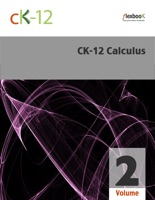# CK-12 Calculus, Volume 2 Book Details

## By CK-12 Foundation

• Category: Mathematics, Books, Science & Nature
• Type: ebook
• Release Date: 2010-11-04
• Author: CK-12 Foundation
• Book Ratings: 3.5/5 (43 User Reviews)
Score Ratings: 3.5
3.5
Based on 43 User Reviews

Get a Copy :

...

### CK-12 Calculus, Volume 2 Overview

CK-12 Foundation’s Calculus, Volume 2 FlexBook covers the following four chapters:
Applications of Integration – This chapter includes applications of the definite integral, such as calculating areas between two curves, volumes, length of curves, and other real-world applications in physics and statistics.
Transcendental Functions – This chapter includes differentiation and integration of logarithmic and exponential functions, exponential growth and decay, derivatives and integrals involving inverse trigonometric functions, and L’Hospital’s Rule.
Integration Techniques – Students explore integration by substitution, integration by parts, integration by partial fractions, trigonometric integrals, trigonometric substitutions, and improper integrals.
Infinite Series – This chapter introduces the study of sequences and infinite series. The properties presented describe the behavior of a sequence or series, including whether a sequence approaches a number or an infinite series adds to a number.